This vignette describes a typical use case of the `MTA` package with a concrete example: the analysis of income inequalities in the Greater Paris Metropolitan Area. This example plays all the functions of the MTA package with appropriate visualizations (plots, maps).

# 1. Introducing case-study and MTA

## 1.1 Multiscalar Territorial Analysis for Policy Study

Beginning in the MTA conceptual framework, several issues must be considered: the study area, the territorial hierarchy and the selected indicator.

• Metropolis of Greater Paris (MGP) : a study area making sense in a political context.
The MGP has been created the 1st January 2016 after a preparatory mission and aims at finding solutions to improve and develop the potential of the Paris metropolitan area to be more present at international level (following the initiatives that took place in London, New-York or Tokyo). The official website (last visit: 2020-12) of this infrastructure provides all the details of this new level of governance. Namely, this spatial planning project proposes to “develop a stronger solidarity between territories, reduce territorial inequalities and propose a rebalancing in term of access to household, employment, training, services and amenities”. For this emerging zoning of spatial planning, the need for indicators and analysis of territorial inequalities is high. The MTA methodology seems to be particularly adapted to propose territorial evidences in this context.

• From the IRIS to the MGP, a lot of hierarchical territorial divisions are available.
The lowest territorial level existing in France associated with public statistical information is IRIS (Ilots Regroupés pour l'Information Statistique). The reference size targeted for each IRIS is 2000 inhabitants (last visit: 2020-12). Each municipality over 10 000 inhabitants and a high proportion of municipalities between 5 000 and 10 000 inhabitants are split in IRIS. It is possible to merge these IRIS in municipalities (36 000 territorial units in France). These municipalities can also be aggregated in Établissements Publics Territoriaux(equivalent to Établissement de Coopération intercommunale, EPCI) or in départements. Each territorial context has specific competences in spatial planning term. Thus, the MGP is constituted by a large number of territorial divisions. It allows the calculation of a high number of deviations according to these territorial contexts. *This analysis is mainly focused on *municipalities and Établissements Publics Territoriaux (EPT) ** but could be extended to other territorial levels.

• Indicators relevant at this scale of analysis.
The chosen indicator (average income tax reference per households) is one of the key drivers of spatial planning policies at local level in France. It is one of the indicators considered relevant for city policy (Politique de la Ville, last visit: 2019-10).

This vignette proposes some concrete outputs for improving the knowledge on income inequalities and proposing solutions for a better geographical distribution of wealth in the MGP area. Relevant statistics will be computed using MTA functionalities and plotted on graphics and maps.

## 1.2 Dataset and complementary Packages

The example dataset, `GrandParisMetropole` includes all the required information to create

• com is a sf object. It includes the geometries, identifiers (DEPCOM) and names (LIBCOM) of the 150 MGP municipalities identifiers and names of the EPT of belonging of each municipality (EPT and LIB_EPT), identifier of the département of belonging of each municipality (DEP) and the statistical indicators for the multiscalar inequality analysis, the numerator (amount of income tax references in Euros, INC) and the denominator (number of tax households, TH) (see ?com).

• EPT is a sf object. It includes the geometries of the 12 EPT of the MGP (see ?EPT).

• cardist is a matrix including the a time distance matrix between municipalities computed using `osrm` package (see ?cardist).

Two additional packages are used: `cartography` for thematic mapping purposes and `ineq` for computing inequality indexes and Lorenz Curve Plot.

``````# load packages
library(MTA)
library(cartography)
``````
``````## Loading required package: sf
``````
``````## Linking to GEOS 3.7.1, GDAL 3.1.2, PROJ 7.1.0
``````
``````##
## Since version 3.0.0 several replacement function have been introduced.
## You can use `tc_legacy_mode('on')` to suppress deprecation warnings.
``````
``````library(ineq)
library(reshape2)
library(sf)

data("GrandParisMetropole", package = "MTA")
# set row names to municipalities names
row.names(com) <- com\$LIBCOM
``````

## 1.3 Context Maps

Context maps highlight the territorial organization of the study area and provide some first insights regarding the spatial patterns introduced by the indicator used for the analysis.

### 1.3.1 Study Area

The 150 municipalities of the MGP belongs to 12 intermediate territorial divisions: the Établissements Publics Territoriaux (EPT). This territorial zoning follows approximately the delineation of the départements : Seine-Saint-Denis (in blue on the map below); Paris (in purple); Hauts-de-Seine (in green) and _Val-de-Marine (in orange).

``````# set margins
par(mar = c(0, 0, 1.2, 0))

# label management
com\$LIBEPT2 <- com\$LIBEPT
com[com\$LIBEPT == "Val de Bievres - Seine Amond - Grand Orly", "LIBEPT2"] <-
"Val de Bievres -\nSeine Amond - Grand Orly"
com[com\$LIBEPT == "Plaine Centrale - Haut Val-de-Marne - Plateau Briard", "LIBEPT2"] <-
"Plaine Centrale -\nHaut Val-de-Marne - Plateau Briard"
com[com\$LIBEPT == "Association des Communes de l'Est Parisien", "LIBEPT2"] <-
"Association des Communes\nde l'Est Parisien"

# label order
epts <- c("Paris",
"Est Ensemble",
"Grand-Paris Est",
"Territoire des aeroports",
"Plaine Commune",
"Boucle Nord 92",
"La Defense",
"Grand Paris Sud Ouest",
"Sud Hauts-de-Seine",
"Val de Bievres -\nSeine Amond - Grand Orly",
"Plaine Centrale -\nHaut Val-de-Marne - Plateau Briard",
"Association des Communes\nde l'Est Parisien")

# colors
colsEPT <- c("#bc60b7", carto.pal("blue.pal", 8)[c(2, 3, 4, 5)],
carto.pal("green.pal", 8)[c(2, 3, 4, 5)],
carto.pal("red.pal", 8)[c(3, 4, 5)])

# zoning
typoLayer(x = com,
var="LIBEPT2", legend.values.order = epts,
legend.pos = "left",
col = colsEPT,
lwd = 0.2,
border = "white",
legend.title.txt = "EPT of belonging")

plot(ept\$geometry, col = NA, border = "black", add = TRUE)

# layout
layoutLayer(title = "Territorial Zoning of the MGP",
sources = "GEOFLA® 2015 v2.1, Apur, impots.gouv.fr",
north = TRUE, scale = 5, col = "white", coltitle = "black",
author = "Ronan Ysebaert, RIATE, 2021")
``````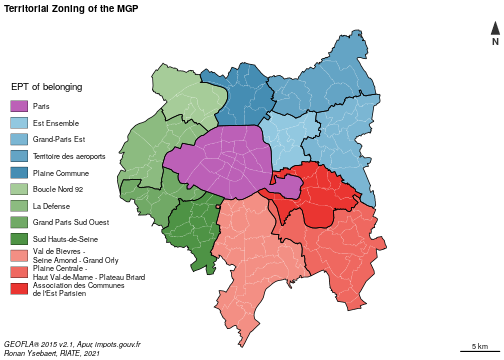### 1.3.2 Map numerator and denominator

The numerator (number of tax households) and the denominator (total amount of income tax references) building the reference ratio (average income per tax households) are firstly plotted.

``````# layout
par(mfrow = c(1, 2), mar = c(0, 0, 1.2, 0))

# numerator map
com\$INCM <- com\$INC / 1000000
plot(com\$geometry,  col = "peachpuff",  border = NA)
plot(st_geometry(ept),  col = NA,  border = "black", add = TRUE)

propSymbolsLayer(x = com,
var = "INCM",
symbols = "circle", col =  "#F6533A",
inches = 0.15,
border = "white",
legend.pos = "topleft", legend.values.rnd = 0,
legend.title.txt = "Amount of income taxe reference\n(millions of euros)",
legend.style = "c")
# layout
layoutLayer(title = "Numerator - Amount of income tax reference",
sources = "GEOFLA® 2015 v2.1, Apur, impots.gouv.fr",
north = FALSE, scale = FALSE, col = "white", coltitle = "black",
author = "Ronan Ysebaert, RIATE, 2021")

# denominator map
plot(st_geometry(com),  col = "peachpuff",  border = NA)
plot(st_geometry(ept),  col = NA,  border = "black", add = TRUE)
propSymbolsLayer(x = com,
var = "TH",
symbols = "circle", col =  "#515FAA",
border = "white",
inches = 0.15,
legend.pos = "topleft", legend.values.rnd = -2,
legend.title.txt = "Number of tax households",
legend.style = "c")

# layout
layoutLayer(title = "Denominator - Tax households",
sources = "", north = TRUE, scale = 5,
col = "white", coltitle = "black")
``````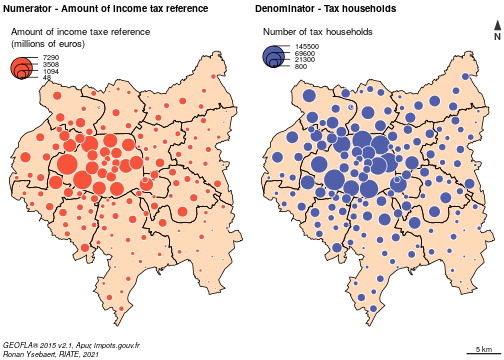Without surprise, the highest amounts of tax households and income are located in the central area of the MGP (Parisian Arrondissements). That being said, these two maps suggest an unequal distribution of income when looking at the distribution of population in Paris suburbs.

### 1.3.3 Ratio

``````par(mar = c(0, 0, 1.2, 0), mfrow = c(1,1))

# ratio
com\$ratio <- com\$INC / com\$TH

# ratio map
choroLayer(x = com,
var = "ratio",
breaks = c(min(com\$ratio, na.rm = TRUE), 20000, 30000, 40000, 50000, 60000,
max(com\$ratio, na.rm = TRUE)),
col = carto.pal(pal1 = "red.pal", n1 = 6),
border = "white",
lwd=0.2,
legend.pos = "topleft",
legend.title.txt = "Average amount of income tax\nreference per households\n(in euros)",
legend.values.rnd = 0)

# EPT borders
plot(ept\$geometry, border = "black",add = TRUE)

# Label min and max
labelLayer(x = com[which.min(com\$ratio),], txt = "LIBCOM", col= "black", cex = 0.6, font = 2,
halo = TRUE, bg = "white", r = 0.05)
labelLayer(x = com[which.max(com\$ratio),], txt = "LIBCOM", col= "black", cex = 0.6, font = 2,
halo = TRUE, bg = "white", r = 0.05)

# layout
layoutLayer(title = "Ratio - Income per tax households, 2013",
sources = "GEOFLA® 2015 v2.1, Apur, impots.gouv.fr",
north = TRUE, scale = 5, col = "white", coltitle = "black",
author = "Ronan Ysebaert, RIATE, 2021")
``````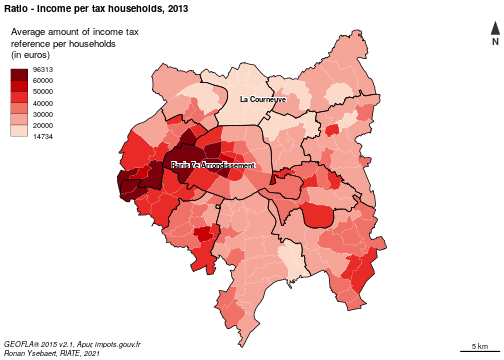The MGP area is characterized by high income inequalities. For the 150 municipalities of this area, the values extend from 14 730 (La Courneuve) to 96 310 euros (Paris_7th Arrondissement). 53 municipalities of the MGP area (35 % of the municipalities) are below the French average, i.e. 25 660 euros. The lagging households are mainly concentrated into the north part of MGP area. Highest values are concentrated in the Western part of Paris and its suburbs.

## 1.4 Introducing the MTA Functions

### 1.4.1 General, territorial and spatial deviations

`MTA` package introduces three contexts to monitor territorial inequalities: the general, the territorial and the spatial deviations.

The global deviation is dedicated to the analysis of inequalities using a value of reference. In this example the global deviation refers to the inequalities existing between each municipality in regard to the whole MGP average.

The territorial deviation consists in measuring the inequalities existing for each basic territorial unit as regards to an intermediate territorial level of reference. In this case-study, it will be the EPT of belonging of each municipality. It implies to include beforehand in the input dataset a territorial level of belong for each territorial unit.

The spatial deviation is a measure of inequalities taking into account the neighborhood as a reference. It allows to measure the deviation existing between basic territorial units using three possible parameters : the territorial contiguity (order 1, 2 or n), the spatial neighborhood (territorial units located at less than X kilometers) or functional distances (territorial units located at less than Y minutes by road for instance). In `MTA`, territorial contiguity and spatial neighborhood measures are directly calculated. Functional distances must be uploaded separately through a dataframe structured with the following fields : id1, id2, distance measure. For this case-study, the contiguity criteria (order 1) will be used for the calculation of the spatial deviation. It gives a good proxy of proximity relationships according to the size and the homogeneity of the municipalities of the MGP area. Other measures could be also adapted, such as time-distances by road (municipalities located at less than 15 minutes by car) or by public transportation.

### 1.4.2 Relative and absolute deviations

In MTA, two methods are implemented to measure statistical differences to a given context of reference: a relative deviation and an absolute deviation. In `MTA`, each indicator is considered as a ratio defined by a numerator (GDP for instance) divided by a denominator (population for instance).

The relative deviation states the position of each region as regards to a reference context. It is based on the following calculation: Relative deviation (Region i) = 100 * ((Numerator(Region i)/Denominator(Region i)) /(reference ratio)) Territorial units characterized by a context of reference below index 100 are under the average of a given reference context, and reciprocally.

The absolute deviation specifies which process of redistribution should be realized in absolute terms to achieve a perfect equilibrium for the ratio of reference in the global, the territorial or the spatial context. It is calculated as below: Absolute deviation (Region i) = Numerator (Region i) - (reference ratio * denominator (Region i)) In concrete terms, it examines how much amount of the numerator should be moved in order to reach equidistribution, for each territorial unit, taking into account as a reference the selected deviation context value. More generally, absolute deviation must be considered as a statistical tool to discuss on the amplitude of existing territorial inequalities. It is obvious that reaching a perfect equilibrium between territorial units is highly sensitive and is scarcely a policy objective itself. It is nevertheless interesting to consider the amount of money or population affected by the inequality to have in hand a concrete material for leading discussions on this delicate spatial planning issue.

### 1.4.3 Cartography of relative and absolute deviations

In this vignette, color palette proposed for displaying on map the relative deviations are the ones suggested by the HyperAtlas tool (blue palette = under the average; red palette = above the average). But other diverging palettes (green/red, etc.) could be also be used for displaying MTA results on maps.

Absolute deviations are highlighted using proportional circles on maps. It examines which amount of the numerator should be moved to the poorest municipalities to reach equidistribution. Circles displayed in a red means that the territorial unit have to contribute a given amount of numerator to achieve the equilibrium in a given context; and reciprocally blue circles means that the territorial unit have to receive frmo the others.

It is quite easy to reproduce or adapt these maps using the functions proposed by the `cartography` package.

### 1.4.4 Synthesis

The analysis of the three deviations provides generally a lot of information. The synthesis functions `bidev` and `mst` helps to summarize values of the global, territorial and spatial deviations and allow to answer to some basic and interesting questions:

• Which territorial units are positioned in a favorable/lagging situation for 2 or 3 deviations?
• Which territorial units are positioned in a contradictory situations ? This is especially interesting from a social and policy points of view (NIMBY phenomena, characterizing the opposition by residents to a proposal for a new development because it is close to them).

Moreover, some additional functions are implemented to ease the mapping (`map_bidev` and `map_mst`) or proposing appropriate plots (`plot_bidev` and `plot_mst`) for visualizing these results.

# 2. Relative deviations

This section aims at describing how computing MTA relative deviations and creating appropriate maps and plots.

## 2.1 Global deviation

First of all, each territorial unit is compared to the overall study area average (Métropole du Grand Paris).

The code below takes in entry the numerator (INC) and the denominator (TH) of the `com` object and returns the global deviation indicators using `gdev()` function. The relative deviation (`type = "rel"`) is computed. This indicator is afterwards mapped.

``````# general relative deviation
com\$gdevrel <- gdev(x = com,
var1 = "INC",
var2 = "TH",
type = "rel")

# margins
par(mar = c(0, 0, 1.2, 0))

# Global colors for deviation
cols <- carto.pal(pal1 = "blue.pal", n1 = 3,  pal2 = "wine.pal", n2 = 3)

# Global deviation mapping
choroLayer(x = com,
var = "gdevrel",
col = cols,
breaks = c(min(com\$gdevrel, na.rm = TRUE),
67, 91, 100, 125, 150,
max(com\$gdevrel, na.rm = TRUE)),
border = "#f0f0f0",
lwd = 0.25,
legend.pos = "topleft",
legend.title.txt = "Deviation to the global context (100 = Metropole du Grand Paris average)",
legend.values.rnd = 0)

# Plot EPT layer
plot(ept\$geometry, border = "#1A1A19", lwd = 1, add = TRUE)

# layout
layoutLayer(title = "Global deviation - Tax income per households",
sources = "GEOFLA® 2015 v2.1, Apur, impots.gouv.fr",
north = TRUE, scale = 5, col = "white", coltitle = "black",
author = "Ronan Ysebaert, RIATE, 2021")
``````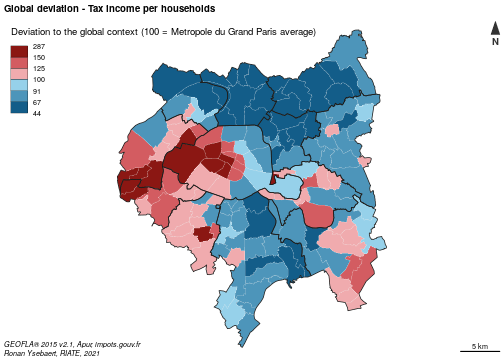The resulting map highlights strong inequalities: First, it is intersting to know that all the municipalities of the EPT of Plaine Commune, Territoire des Aéroports and Est Ensemble are below the average of the MGP area (33 501 euros per households). For the territories of Val de Bièvres and Grand-Paris Est, only three municipalities are above the average of the study area. Reversely, all the municipalities of the Grand-Paris-Sud-Ouest EPT are largely above the average of the MGP area. For the other EPT the situation is mixed, depending of the municipalities.

The `ineq` package proposes some functions to depict global inequalities existing in a study area, such as the Lorenz-curve. The `Lc` function takes in entry the numerator and the denominator and returns a Lorenz Curve plot; inequality indexes take in entry the ratio (numerator / denominator) and returns econometric indexes of inequality.

The code below add some additional graphical parameters to ease the interpretation of this plot.

``````library(ineq)
#  Concentration of X as regards to concentration of Y
Lc.p <- Lc(com\$INC, com\$TH)
Lp <- data.frame(cumX = 100 * Lc.p\$L, cumY = 100 * Lc.p\$p)

# Plot concentrations
par(mar = c(4,4,1.2,4), xaxs = "i", yaxs = "i", pty = "s")
plot(Lp\$cumY,
Lp\$cumX,
type = "l",
col = "red",
lwd = 2,
panel.first = grid(10,10),
ylab = "Income (cumulative percentage)",
cex.axis = 0.8,
cex.lab = 0.9,
xlab = "Households (cumulative percentage)",
ylim = c(0,100),
xlim = c(0,100))

lines(c(0,100), c(0,100), lwd = 2)

xy1 <- Lp[which.min(abs(50 - Lp\$cumX)),]
xy2 <- Lp[which.min(abs(50 - Lp\$cumY)),]

xy <- rbind(xy1, xy2)

points(y = xy[,"cumX"],
x = xy[,"cumY"],
pch = 21,
cex = 1.5,
bg = "red")

text(y = xy[,"cumX"],
x = xy[,"cumY"],
label = paste(round(xy[,"cumX"],0), round(xy[,"cumY"],0), sep = " , "),
pos = 2,
cex = 0.6)
``````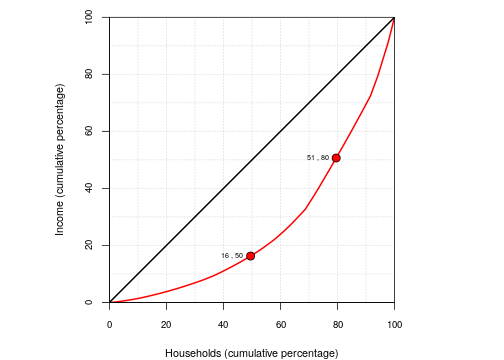The curve depicts on its horizontal axis a defined population – e.g., all households – broken down into deciles and ordered from left to right on the horizontal axis (from the lower tax income per household to the higher). On the vertical axis of the Lorenz curve is shown the cumulative percentage of tax income.

The reading of the plot reveals these following configurations :

• 50 % of the households earns less than 16 % of the total income.
• 50 % of the total income is held by less than 20 % of households.

## 2.2 Territorial deviation

The territorial deviation is now calculated to analyze the position of each municipality as regards to a territorial level of reference: the (EPT) of belonging in this case-study.

The `tdev` function takes in entry the numerator (INC) and the denominator (TH) of the `com` object. The territorial level of belonging in specified with the `key` argument. The territorial relative deviation is returned by the function.

``````# Territorial relative deviation calculation
com\$mdevrel <- tdev(x = com,
var1 = "INC",
var2 = "TH",
type = "rel",
key = "LIBEPT")

# Cartography
# Plot layout
par(mfrow = c(1, 1), mar = c(0, 0, 1.2, 0))

# Territorial deviation mapping
choroLayer(x = com,
var = "mdevrel",
col = cols,
breaks = c(min(com\$mdevrel, na.rm = TRUE), 67, 91, 100, 125, 150,
max(com\$mdevrel, na.rm = TRUE)),
border = "#f0f0f0",
lwd = 0.25,
legend.pos = "topleft",
legend.title.txt = "Deviation to the territorial context (100 = EPT average)",
legend.values.rnd = 0)

# Plot EPT layer
plot(ept\$geometry, border = "#1A1A19", lwd = 1, add = TRUE)

# Labels to ease comment location
labelLayer(x = com[com\$LIBCOM %in% c("Le Raincy", "Rungis", "Sceaux",
"Marnes-la-Coquette") ,],
txt = "LIBCOM", col= "black", cex = 0.6, font = 2,
halo = TRUE, bg = "white", r = 0.05)

# layout
layoutLayer(title = "Territorial deviation - Tax income per households, 2013",
sources = "GEOFLA® 2015 v2.1, Apur, impots.gouv.fr",
north = TRUE, scale = 5, col = "white", coltitle = "black",
author = "Ronan Ysebaert, RIATE, 2021")
``````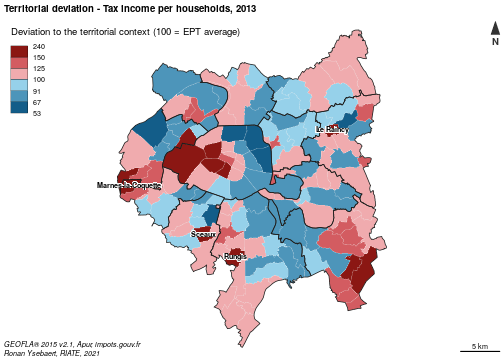This map highlights existing inequalities in each EPT: The strongest differences in relative terms are located in Paris (opposition between the eastern part and the western part of this EPT) and in the Plaine centrale - Haut Val de Marne EPT (opposition between the poorest municipalities located near Paris and the ones located in the periphery). Globally, the richest and the poorest EPT ( Grand Paris Sud Ouest / Plaine Commune and Territoires des Aéroports du Nord Ouest) appear relatively homogeneous statistically. In other EPT, one municipality appears largely above the average of their EPT of belonging. It is namely the case in Grand-Paris-Sud-Ouest (Marnes-la-Coquette), Sud Hauts-de-Seine (Sceaux), Grand Orly Seine Biève (Rungis) or Grand Paris Grand Est (Le Raincy)

Another way to explore characteristics of the territorial deviation consists in analyzing the statistical dispersion (general deviation) by intermediate level (EPT in this case). The best suited graphical representation for this is certainly a boxplot.

The code below takes in entry the general deviation calculated above and the intermediate levels included in the input dataset. It returns a boxplot displaying the statistical parameters (median, mean, 1st and 3rd quartiles, range, minimum and maximum, extraordinary values) allowing to observe the statistical dispersion existing for each intermediate zoning. To ease the interpretation and the synthesis of the plot, boxplots are ordered by the average of each territorial level. Moreover, the width of the bars are proportional to the number of territorial units included in each intermediate zoning.

``````par(mar = c(4, 4, 1.2, 2))

# Drop geometries
df <- st_set_geometry(com, NULL)

# Reorder EPT according to gdev value
df\$EPT <- with(df, reorder(EPT, gdevrel, mean, na.rm = TRUE))

# Colors management
col <- carto.pal(pal1 = "red.pal", n1 = (nlevels(df\$EPT) / 2),
pal2 = "green.pal", n2 = (nlevels(df\$EPT) / 2),
middle = FALSE, transparency = TRUE)

# Boxplot
boxplot(df\$gdevrel ~ df\$EPT,
col = col,
ylab = "Global deviation",
xlab = "Territorial deviation",
cex.lab = 0.9,
varwidth = TRUE,
range = 1,
outline = TRUE,
las = 1)

# Horizontal Ablines
abline (h = seq(40, 300, 10), col = "#00000060", lwd = 0.5, lty = 3)

# Plot mean values
xi<- tapply(df\$gdevrel, df\$EPT, mean, na.rm = TRUE)
points(xi, col = "#7C0000", pch = 19)

# Legend for the boxplot
df\$EPTName<- as.factor(df\$LIBEPT)
df\$EPTName <- with(df, reorder(EPTName, gdevrel, mean, na.rm = TRUE))
legend("topleft",
legend = levels(df\$EPTName),
pch = 15,
col = col,
cex = 0.6,
pt.cex = 1,
title = "Territorial contexts (ordered by mean value of global deviation)")
``````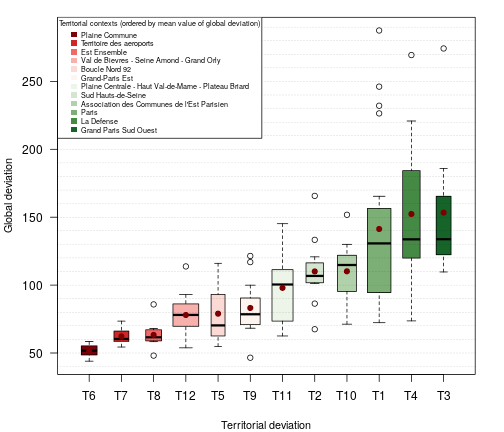This plot highlights the statistical dispersion existing in each EPT. It confirms globally that wealthier the EPT is, larger the statistical differences between the poorest and the wealthiest territorial units are. In that way, Plaine Commune and Territoire des Aéroports (T6 and T7) appear quite homogeneous in a lagging situation. On the opposite, important differences a revealed in Paris (minimum = 71 and maximum = 290) or in La Defense and Grand Paris Sud Ouest.

The boxplot highlights also outliers (dots out of the box) in each territorial context. Most EPT are concerned: wealthiest EPT (Paris, La Défense, Grand Paris Sud Ouest and ACEP, generally characterized by high outliers) and EPT in less favorable situation (Est Ensemble, Grand Paris Est and Sud-Hauts-de-Seine, which include municipalities with include both low and high outliers.

## 2.3 Spatial deviation

The spatial deviation is calculated to position territorial units in a neighborhood context. Several criteria may be considered (geographical distance, time-distance). In this example, each municipality will be compared to the average of contiguous municipalities (contiguity order 1).

The `sdev` function takes in entry the numerator (INC) and the denominator (TH) of the `com` object. The contiguity order 1 is set in the `order` argument.

``````# Spatial relative deviation calculation
com\$ldevrel <- sdev(x = com,
xid = "DEPCOM",
var1 = "INC",
var2 = "TH",
order = 1,
type = "rel")

# Cartography
# Plot layout
par(mfrow = c(1, 1), mar = c(0, 0, 1.2, 0))

# Territorial deviation (relative and absolute) cartography
choroLayer(x = com,
var = "ldevrel",
col = cols,
breaks = c(min(com\$ldevrel, na.rm = TRUE), 67, 91, 100, 110, 125,
max(com\$ldevrel, na.rm = TRUE)),
border = "#f0f0f0",
lwd = 0.25,
legend.pos = "topleft",
legend.title.txt = "Deviation to the spatial context\n(100 = average of the contiguous territorial units - order 1)",
legend.values.rnd = 0)

# Plot EPT
plot(ept\$geometry, border = "#1A1A19",lwd = 1, add = T)

# Labels to ease comment location
labelLayer(x = com[com\$LIBCOM %in% c("Le Raincy", "Vaucresson", "Sceaux",
"Marnes-la-Coquette", "Saint-Maur-des-Fosses",
"Puteaux", "Saint-Ouen", "Bagneux",
"Clichy-sous-Bois", "Clichy") ,],
txt = "LIBCOM", col= "black", cex = 0.6, font = 2,
halo = TRUE, bg = "white", r = 0.05, overlap = FALSE)

# layout
layoutLayer(title = "Spatial deviation - Tax income per households, 2013",
sources = "GEOFLA® 2015 v2.1, Apur, impots.gouv.fr",
north = TRUE, scale = 5, col = "white", coltitle = "black",
author = "Ronan Ysebaert, RIATE, 2021")
``````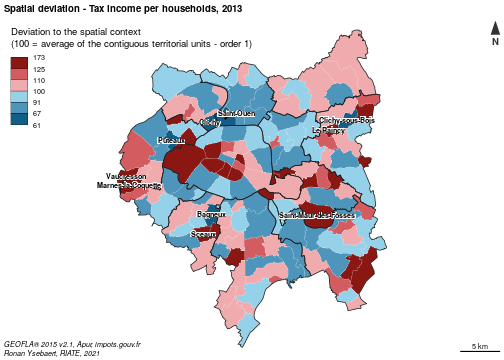This map highlights local discontinuities existing in the study area. Important local statistical gaps appear in several areas: Saint-Mandé and Neuilly-sur-Seine are characterized by the highest score as regards to their respective neighbors (indexes 173 and 156).Some local “bastions” are revealed in Paris suburbs, such as Le Raincy (index 153), Sceaux (150), Vaucresson (143), Marnes-la-Coquette (142) or Saint-Maur-des-Fossés (140).

On the reverse situation, lower index are observed at Clichy-sous-Bois. Clichy, Puteaux, Saint-Ouen and Bagneux. Their average income per household is around 30-40 % below their respective neighborhood.

Spatialized indicators are often subject to spatial dependencies (or interactions), which are even stronger than spatial locations are closer. Autocorrelation measures (Moran, Geary, Lisa indexes) allows estimating the spatial dependence between the values of a same indicator at several locations of a given study area.

The figure displayed below consists in evaluating this spatial autocorrelation by a plot crossing the spatial deviation (Y axis) and global deviation (X axis) values.

This plot provides interesting inputs for answering to basic questions, such as:

• Are territorial units in favorable situation in a global context also in the same situation at local level?
• Is it possible to detect specific situations as regard to the global trend, meaning the existence of “local bastions” in favorable or lagging situations?
``````par(cex.lab = 1, cex.axis = 0.75, mar = c(4, 4, 1.2, 2))

# Drop geometries
df <- st_set_geometry(com, NULL)

# label order
df\$LIBEPT <- as.factor(df\$LIBEPT)
df\$LIBEPT <- ordered(df\$LIBEPT,
levels = c("Paris",
"Est Ensemble",
"Grand-Paris Est",
"Territoire des Aéroports",
"Plaine Commune",
"Boucle Nord 92",
"La Defense",
"Grand Paris Sud Ouest",
"Sud Hauts-de-Seine",
"Val de Bievres - Seine Amond - Grand Orly",
"Plaine Centrale - Haut Val-de-Marne - Plateau Briard",
"Association des municipalities de l'Est Parisien"))

# colors
df\$col <- as.factor(df\$LIBEPT)
levels(df\$col) <- colsEPT

# Spatial autocorrelation
lm <- summary.lm(lm(ldevrel ~ gdevrel, df))

# Plot spatial autocorrelation
plot(df\$gdevrel, df\$ldevrel,
ylab = "Local deviation",
ylim = c(50,260),
xlab = "Global deviation",
xlim = c(50,260),
pch = 20,
col = as.vector(df\$col),
asp = 1)
abline((lm(df\$ldevrel ~ df\$gdevrel)), col = "red", lwd =1)

# Specify linear regression formula and R-Squared of the spatial autocorrelation on the plot
text(110,60, pos = 4, cex = 0.7,
labels = (paste("Local Deviation =", round(lm\$coefficients["gdevrel","Estimate"], digits = 3),
"* (Global Deviation) +", round(lm\$coefficients["(Intercept)","Estimate"],
digits = 3))))
text(110,55, pos = 4, cex = 0.7,
labels = (paste("R-Squared =", round(summary(lm(ldevrel~gdevrel, com ))\$r.squared, digits = 2))))

abline (h = seq(40,290,10), col = "gray70", lwd = 0.25, lty = 3)
abline (h = seq(50,250,50), col = "gray0", lwd = 1, lty = 1)
abline (v = seq(40,290,10), col = "gray70", lwd = 0.25, lty = 3)
abline (v = seq(50,250,50), col = "gray0", lwd = 1, lty = 1)

# Legend for territorial level
legend("topleft",
legend = levels(df\$LIBEPT),
pch = 20,
col = colsEPT,
cex = 0.6,
pt.cex = 1,
title = "Territorial context")
``````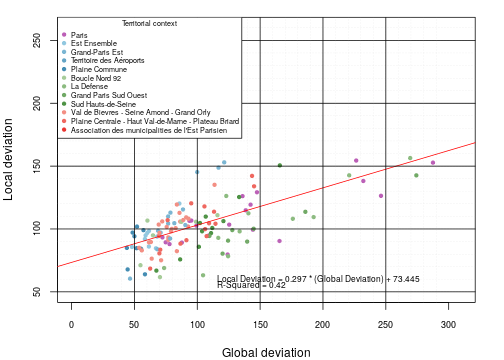The output of the linear model of spatial autocorrelation reveals that the hypothesis of independence is rejected at a probability below than 0.0001. It means that, “everything is related to everything else, but near things are related than distant things” (Tobler, 1970). However, the R-squared of the relation (0.42) suggests that this statistical relation includes outliers very far from the linear regression.

This chart can be modified for analyzing outliers specifically. The code below computes the statistical residuals of the spatial autocorrelation calculated above. Then the residuals are standardized. Finally, a plot is built to display and label significant residuals.

``````par(cex.lab = 1, cex.axis = 0.75, mar = c(4, 4, 2, 2))

# Standardized residual calculation
lm <- lm(ldevrel ~ gdevrel, df)
df\$res <- rstandard(lm)

#risk alpha (0.1 usually)
alpha <- 0.055

# Calculation of the threshold using T-Student at (n-p-1) degrees of freedom
thr <- qt(1 - alpha / 2, nrow(com) - 1)

# Plot residuals
plot(df\$ldevrel, df\$res,
xlab = "Local deviation", cex.lab = 0.8,
ylim = c(-3.5, 3.5),
xlim = c(40, 200),
ylab = "Standardized residuals of spatial autocorrelation",
cex.lab = 0.8,
cex.axis = 0.8,
pch = 20,
col = as.vector(df\$col))

abline(h = - thr, col = "red")
abline(h = + thr, col = "red")
abline(h = 0, col = "red")

# Detecting exceptional values and labeling them on the plot
ab <- df[df\$res < -thr | df\$res > thr,]

# Plot residual labels
text(x = ab[,"ldevrel"], y = ab[,"res"], ab[,"LIBCOM"], cex = 0.5, pos = 4)

abline (v = seq(50, 200, 10), col = "gray70", lwd = 0.25, lty = 3)
abline (v = seq(50, 200, 50), col = "gray0", lwd = 1, lty = 1)

# Plot the legend (territorial zoning)
legend("topleft",
legend = levels(ab\$LIBEPT),
pch = 20,
col = colsEPT,
cex = 0.6,
pt.cex = 1,
title = "Territorial context")
``````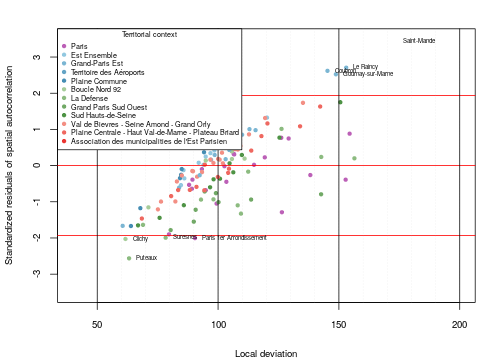Further analysis may be considered in the domain, using for instance Moran indexes et LISA indexes or other distance criteria. This, it is important to remind that the choice of the appropriate threshold or criteria must make sense from a thematic point of view (does a neighborhood of 5 km mean something? Is a distance of 20 minutes by car more appropriated?)

# 3. Redistributions

Redistributions highlight another way to analyze territorial disparities. The analysis is no more focused on inequalities themselves, but rather than to the statistical process (amount of numerator or denominator) required to reach the equilibrium in each territorial context.

The meaning of this mathematical calculation is strong in policy term, since it point out the municipalities which may have to contribute / receive the highest to ensure a perfect equidistribution in several territorial contexts. It gives also the amount of the reallocation process.

The calculation is made for the general and the territorial contexts, using the argument `type = abs`. Two maps are created, displaying the amount of income that should be transfered from the wealthiest to the poorest municipalities.

``````# general absolute deviation
com\$gdevabs <- gdev(x = com,
var1 = "INC",
var2 = "TH",
type = "abs")

# Territorial absolute deviation calculation
com\$mdevabs <- tdev(x = com,
var1 = "INC",
var2 = "TH",
type = "abs",
key = "LIBEPT")

# Transform the values in million Euros
com\$gdevabsmil <- com\$gdevabs / 1000000
com\$mdevabsmil <- com\$mdevabs / 1000000

# Deviation orientation
com\$gdevsign <- ifelse(com\$gdevabsmil> 0, "Income surplus", "Income deficit")
com\$mdevsign <- ifelse(com\$mdevabsmil > 0, "Income surplus", "Income deficit")

# Deviation maps
par(mar = c(0, 0, 1.2, 0), mfrow = c(1,2))

# General deviation
# Plot territories
plot(st_geometry(com), col = "grey70", border = "#EDEDED", lwd = 0.25)
plot(st_geometry(ept), border = "#1A1A19", lwd = 1, add = TRUE)

propSymbolsTypoLayer(x = com, var = "gdevabsmil", var2 = "gdevsign",
symbols = "circle",
inches = 0.15,
col = c("#F6533A","#515FAA"),
legend.var.pos = "n",
legend.var2.pos = "n",
fixmax = max(abs(com\$gdevabsmil)))
``````
``````## Warning: `propSymbolsTypoLayer()` is deprecated as of cartography 3.0.0.
## This warning is displayed once every 8 hours.
## Call `lifecycle::last_warnings()` to see where this warning was generated.
``````
``````# Labels to ease comment location
labelLayer(x = com[com\$LIBCOM %in% c("Paris 7e Arrondissement",
"Neuilly-sur-Seine", "Aubervilliers") ,],
txt = "LIBCOM", col= "black", cex = 0.6, font = 2,
halo = TRUE, bg = "white", r = 0.05, overlap = FALSE)

# Layout map 1
layoutLayer(title = "General deviation (Metrople du Grand Paris)",
tabtitle = TRUE, col = "white",
coltitle = "black", postitle = "center", scale = FALSE,
author = "Ronan Ysebaert, RIATE, 2021")

# Territorial deviation
plot(st_geometry(com), col = "grey70", border = "#EDEDED", lwd = 0.25)
plot(st_geometry(ept), border = "#1A1A19", lwd = 1, add = TRUE)

propSymbolsTypoLayer(x = com, var = "mdevabsmil", var2 = "mdevsign",
symbols = "circle",
inches = 0.15,
col = c("#F6533A","#515FAA"),
legend.var.pos = "n",
legend.var2.pos = "n",
fixmax = max(abs(com\$gdevabsmil)))

# Labels to ease comment location
labelLayer(x = com[com\$LIBCOM %in% c("Marnes-la-Coquette",
"Nanterre", "Clichy-sous-Bois") ,],
txt = "LIBCOM", col= "black", cex = 0.6, font = 2,
halo = TRUE, bg = "white", r = 0.05, overlap = FALSE)

# Legend cirles
legendCirclesSymbols(pos = "topleft", inches = 0.15, col = "grey",
var = c(500, 2000, max(com\$gdevabsmil)),
title.txt = "Income redistribution\n(million Euros)")
``````
``````## Warning: `legendCirclesSymbols()` is deprecated as of cartography 3.0.0.
## This warning is displayed once every 8 hours.
## Call `lifecycle::last_warnings()` to see where this warning was generated.
``````
``````# Legend typo
legendTypo(pos = "bottomleft", title.txt = "Redistribution nature", col = c("#F6533A","#515FAA"),
categ = c("Income surplus", "Income deficit"), nodata = FALSE)
``````
``````## Warning: `legendTypo()` is deprecated as of cartography 3.0.0.
## This warning is displayed once every 8 hours.
## Call `lifecycle::last_warnings()` to see where this warning was generated.
``````
``````# Layout map 2
layoutLayer(title = "Territorial deviation (EPT of belonging)",
tabtitle = TRUE, col = "white",
coltitle = "black", postitle = "center", scale = 5)
``````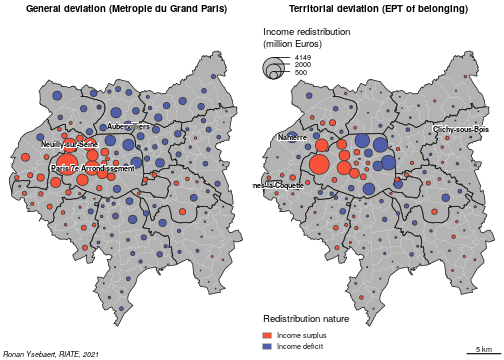In the context of the MGP, the 7th Arrondissement of Paris is the municipality which should contribute the most, all things being equal to its income tax level (1,987 billion Euros of income transfer, 65 % of the total amount of tax income in this municipality). Neuilly-sur-Seine (third position in absolute terms) should transfer 1,9 billion Euros to the lagging municipalities of the MGP area. It corresponds to 62,87% of the total amount of tax income declared in this municipality.

In the other side of the redistribution, La Courneuve should receive 402 million Euros from the wealthiest municipalities (127 % of the current tax income declared). Aubervilliers should receive 793 million Euros, which represents 124 % of the current tax income in this municipality.

The chunks below compute the top 10 theoretical contributors and receivers municipalities (total amount of income and share of current available income) to reach a perfect equilibrium.

``````# general deviation - Top 10 of the potential contributors in regard
# Drop geometries
df <- st_set_geometry(com, NULL)
row.names(df) <- df\$LIBCOM

# to their total amount of income
df\$gdevabsPerc <- df\$gdevabs / df\$INC * 100
df <- df[order(df\$gdevabsPerc, decreasing = TRUE), ]
df[1:10, c("gdevabsmil","gdevabsPerc")]
``````
``````##                          gdevabsmil gdevabsPerc
## Paris 7e Arrondissement  1987.18261    65.21643
## Marnes-la-Coquette         46.58689    63.53843
## Neuilly-sur-Seine        1900.79972    62.87958
## Paris 8e Arrondissement  1167.45176    59.37545
## Paris 16e Arrondissement 4148.92114    56.91599
## Paris 6e Arrondissement  1030.25933    55.83634
## Vaucresson                174.81548    54.71988
## Saint-Cloud               473.60724    48.07040
## Ville-d'Avray             165.60016    46.23143
## Garches                   246.70718    43.19362
``````
``````# general deviation - Top 10 of the potential receivers in regard to
# their total amount of income
df <- df[order(df\$gdevabsPerc, decreasing = FALSE), ]
df[1:10, c("gdevabsmil", "gdevabsPerc")]
``````
``````##                          gdevabsmil gdevabsPerc
## La Courneuve              -402.2023  -127.37566
## Aubervilliers             -793.0032  -124.08051
## Clichy-sous-Bois          -243.2493  -114.90485
## Bobigny                   -465.2779  -108.01736
## Stains                    -313.7436  -105.88273
## Villetaneuse              -104.6940   -99.97363
## Saint-Denis               -941.8914   -93.24860
## Pierrefitte-sur-Seine     -237.5380   -92.02924
## Villeneuve-Saint-Georges  -279.8035   -85.87404
## Dugny                      -81.3603   -83.81888
``````

Looking at the EPT context, the 7th Arrondissement of Paris is still the municipality which should contribute the most to the poorest municipalities of Paris as regards to the amount of income available in this municipality (1,779 billion Euros of income transfer, 58 % of the amount of income in this municipality). Marnes-La-Coquette (second position) should transfer 38 million Euros to the poorest municipalities of its EPT of belonging (La Défense). Despite the low income mass, it corresponds to 52 % of the total amount of income available in this municipality.

From the other side of the redistribution, Nanterre, Clichy-sous-Bois and the 19th Arrondissement of Paris should receive respectively 1088, 143 and 1926 million euros from the wealthiest municipalities of their EPT of belonging (respectively 88 %, 68 % and 65 % of the total amount of earned income of their households). The highest redistribution for this study area stands for the 20th Arrondissement of Paris (1,926 billion Euros, 59 % of its total amount of income).

``````# general deviation - Top 10 of the potential contributors in regard
# Drop geometries
df <- st_set_geometry(com, NULL)
row.names(df) <- df\$LIBCOM

# Territorial deviation - Top 10 of the potential contributors as regards to their total amount of income
df\$mdevabsPerc <- df\$mdevabs / df\$INC * 100
df <- df[order(df\$mdevabsPerc, decreasing = TRUE), ]
df[1:10, c("mdevabsmil", "mdevabsPerc")]
``````
``````##                          mdevabsmil mdevabsPerc
## Paris 7e Arrondissement  1779.21614    58.39127
## Marnes-la-Coquette         37.85668    51.63157
## Paris 8e Arrondissement  1010.71931    51.40419
## Neuilly-sur-Seine        1468.33529    48.57340
## Paris 16e Arrondissement 3532.67330    48.46214
## Paris 6e Arrondissement   870.36502    47.17065
## Santeny                    37.74031    43.38812
## Marolles-en-Brie           47.14636    42.74486
## Vaucresson                119.06443    37.26896
## Le Raincy                 116.35420    35.78738
``````
``````# Territorial deviation - Top 10 of the potential receivers as regards to their total amount of income
df <- df[order(df\$mdevabsPerc, decreasing = FALSE), ]
df[1:10, c("mdevabsmil", "mdevabsPerc")]
``````
``````##                          mdevabsmil mdevabsPerc
## Nanterre                 -1087.5931   -88.08889
## Clichy-sous-Bois          -143.1380   -67.61479
## Paris 19e Arrondissement -1925.8379   -65.44786
## Paris 20e Arrondissement -1958.2712   -59.73389
## Bagneux                   -295.2509   -58.59873
## Paris 18e Arrondissement -1864.1104   -53.46040
## Champigny-sur-Marne       -509.8546   -49.20335
## Gennevilliers             -197.9141   -43.87097
## Villeneuve-Saint-Georges  -123.2206   -37.81744
## Paris 13e Arrondissement -1241.4815   -36.30654
``````

# 4. Synthesis

This section shows the synthesis functions of the `MTA` package: `bidev`, `plot_bidev` and `map_bidev` are useful to synthetize, plot and map the situation for 2 selected relative deviations. The `mst`, `plot_mst` and `map_mst` are suitable for 3 deviations.

## 4.1 Two-relative deviations synthesis

The `bidev` function allows firstly to position each territorial unit as regards to 2 deviations (`dev1` and `dev2`) already calculated; and secondly to evaluate the statistical distance to the average (index 100). This function returns a vector which is the combination of two modalities :

• A: deviations above index 100 for the two selected contexts.
• B: above 100 for `dev1` and below 100 for `dev2`.
• C: below 100 for `dev1` and `dev2`.
• D: below 100 for `dev1` and above 100 for `dev2`.

Are combined to modalities A, B, C and D the distance to the average. By default, it corresponds to 25 %, 50 % and 100 % under-above the average. Adapted to normalized index where 100 is average, it creates 4 classes :

• ZZ : Values below 25 % of the average, corresponding to indexes 80 and 125).
• 1 : Values between 25 % and 50 % of the average, corresponding to indexes 67-80 and 125-150.
• 2 : Values between 50 % and 100 % of the average, corresponding to indexes 50-67 and 150-200.
• 3 : Values above 50 % of the average, corresponding to indexes below 50 and above 200.

At this end, it produces a 13 classes-typology, which is the combination of the two modalities described above (classes A1, B3, C2, etc.). The user is free to modify the breaks proposed by default by the function (`breaks = c(25, 50, 100)`)

To understand the resulting typology, the `plot_mst` is especially adapted. The X-Y scale is defined in logarithm. Index 200 corresponds to territorial units defined to twice the average ; and index 50 twice below the average.

``````par(mar = c(0, 0, 0, 0), mfrow = c(1,1))

# Prerequisite  - Compute 2 deviations
com\$gdev <- gdev(x = com, var1 = "INC", var2 = "TH")
com\$tdev <- tdev(x = com, var1 = "INC", var2 = "TH", key = "EPT")

# EX1 standard breaks with four labels
plot_bidev(x = com,
dev1 = "gdev",
dev2 = "tdev",
dev1.lab = "General deviation (MGP Area)",
dev2.lab = "Territorial deviation (EPT of belonging)",
lib.var = "LIBCOM",
lib.val =  c("Marolles-en-Brie", "Suresnes",
"Clichy-sous-Bois", "Les Lilas"))
``````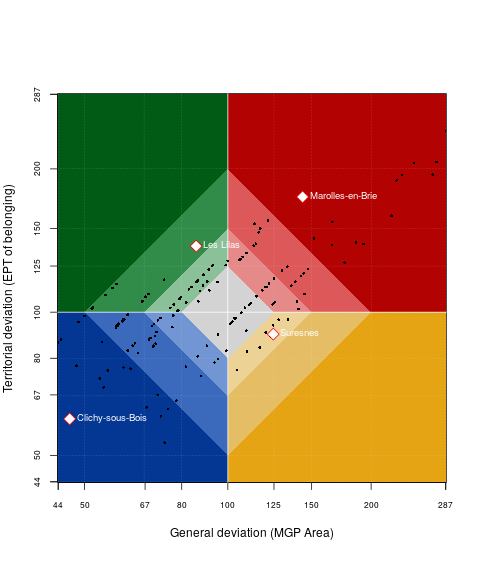This plot highlights specific areas of interest. In the red quarter, territorial units above the average for the general and territorial deviations, such as Marolles-en-Brie(class A3). Reversely, municipalities appearing in the blue quarter are below the average for the 2 deviations, such as Clichy-sous-Bois(class C3).

It shows also territorial units in contradictory situations, such as Les Lilas(class D2, green quarter), which is in lagging situation for the general deviation and in favorable situation for the territorial context ((EPT of belonging). Reversely, Suresnes is in favorable situation in the context of the MGP and in lagging situation in its EPT of belonging.

The `map_bidev` function delivers a list of 3 objects useful to map the results: a `sf` object (`geom`) including the result of the `bidev` function (new column). This object is ordered following the 13 classes. It delivers also a color vector for mapping purpose. It includes only resulting categories: if the dataset does not include “D3” class, the resulting `cols` object will not include dark green color.

For mapping the results, it is recommended to share the plot in two columns : one side for the map, and one side displaying the bidev plot to understand correctly the colors displayed on the map.

``````par(mfrow = c(1,2), mar = c(0,4,0,0))

bidev <- map_bidev(x = com, dev1 = "gdev", dev2 = "tdev",
breaks = c(50, 100, 200))

# Unlist resulting function
com <- bidev\$geom
cols <- bidev\$cols

# Cartography
typoLayer(x = com, var = "bidev", border = "grey50",
col = cols, lwd = 0.2, legend.pos = "n")

plot(st_geometry(ept), border = "#1A1A19", lwd = 1, add = TRUE)

# Label territories in the C3 category
labelLayer(x = com[com\$bidev == "C3",], txt = "LIBCOM", halo = TRUE)

layoutLayer(title = "2-Deviations synthesis : Situation as regards to general and territorial contexts",
sources = "GEOFLA® 2015 v2.1, Apur, impots.gouv.fr",
north = TRUE, scale = 5, col = "white", coltitle = "black",
author = "Ronan Ysebaert, RIATE, 2021")

plot_bidev(x = com,  dev1 = "gdev",  dev2 = "tdev",
dev1.lab = "General deviation (MGP Area)",
dev2.lab = "Territorial deviation (EPT of belonging)",
breaks = c(50, 100, 200),
lib.var = "LIBCOM", lib.val = "Clichy-sous-Bois", cex.lab = 0.8)
``````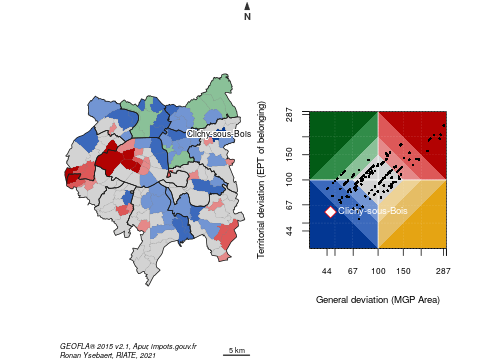This map highlights areas in favorable situation for the MGP area and EPT contexts (red), in lagging situation for these two contexts (blue) or in contradictory situations (yellow and green). Darker is the color, stronger are the distance to the average.

## 4.2 Three-relative deviations synthesis

The three relative deviations (general, territorial, spatial) can be summarized in a synthetic typology using the `mst` function. It allows, according to a pre-defined threshold (100 being the average), to higlight the territorial units which are under or above this threshold, and for which of the 3 deviations proposed by the MTA package.

Assuming (for instance) that the treshold is equal to 100 (value corresponding to the average for each deviations) and the superior argument is false (consider the value below the average), the `mst()` function returns a 8-classes typology (mst column) which can be interpreted as follows:

• Class 7: A territorial unit described by a mst value equal to 7 is under (`superior = FALSE`) the average (`threshold = 100`) for all the deviations.
• Classes 1, 2 and 4 : The territorial unit is under the average for one deviation only (1: general deviation, 2: territorial deviation and 4: spatial deviation).
• Classes 3, 5 and 7: The territorial unit is under the average for 2 of the 3 possible deviations (3: general and territorial deviations, 5: general and spatial deviations and 6: territorial and spatial deviations).
• Class 0: The territorial unit is under the average for none of the deviations, meaning that it is above the average for all the deviations.

As demonstrated below, this typology is especially useful to highlight territories in lagging or favorable situations, but also territories in contradictory situations.

### 4.2.1 Wealthiest municipalities

We focus firtstly the analysis on municipalities above 125 % for the three deviations (gdev = Métropole du Grand Paris ; tdev = EPT average and sdev = contiguous municipalities, threshold = 125, superior = TRUE). The `mst()` function returns their position according to the three deviations. The subset (mst == 7) returns all the municipalities in this situation.

``````# Prerequisite  - Compute the 3 deviations
com\$gdev <- gdev(x = com, var1 = "INC", var2 = "TH")
com\$tdev <- tdev(x = com, var1 = "INC", var2 = "TH", key = "EPT")
com\$sdev <- sdev(x = com, var1 = "INC", var2 = "TH", order = 1)

# Multiscalar typology - wealthiest territorial units
# Row names = municipality labels
row.names(com) <- com\$LIBCOM

# Compute mst
com\$mstW <- mst(x = com, gdevrel = "gdev", tdevrel = "tdev", sdevrel = "sdev",
threshold = 125, superior = TRUE)

subset(com, mstW == 7, select = c(ratio, gdev, tdev, sdev, mstW), drop = T)
``````
``````##                             ratio     gdev     tdev     sdev mstW
## Sceaux                   55513.65 165.7067 154.8138 150.5888    7
## Marolles-en-Brie         48101.68 143.5822 174.6568 142.2428    7
## Saint-Mande              50852.51 151.7934 142.8341 173.4663    7
## Santeny                  48648.24 145.2137 176.6414 133.9427    7
## Paris 6e Arrondissement  75856.82 226.4305 189.2887 154.4339    7
## Paris 7e Arrondissement  96313.13 287.4920 240.3342 152.7925    7
## Paris 8e Arrondissement  82465.28 246.1566 205.7791 126.4014    7
## Paris 16e Arrondissement 77757.72 232.1047 194.0321 138.1793    7
## Marnes-la-Coquette       91880.71 274.2614 206.7464 142.6436    7
## Neuilly-sur-Seine        90249.91 269.3935 194.4519 156.4231    7
## Vaucresson               73986.43 220.8475 159.4107 142.6996    7
``````

The results are afterwards mapped. As `map_bidev` function do, the `map_mst` function takes in entry the 3 pre-calculated deviations and `mst` parameters and returns a list including a `sf` object with the result of the `mst` calculation, and two vectors proposing colors and legend for mapping results.

``````par(mfrow = c(1, 1), mar = c(0, 0, 1.2, 0))

# Compute mapmst
mst <- map_mst(x = com, gdevrel = "gdev", tdevrel = "tdev", sdevrel = "sdev",
threshold = 125, superior = TRUE)

# Unlist resulting function
com <- mst\$geom
cols <- mst\$cols
leg_val <- mst\$leg_val

# Cartography
library(cartography)
typoLayer(x = com, var = "mst", border = "grey50",
col = cols, lwd = 0.2, legend.pos = "n")

plot(st_geometry(ept), border = "#1A1A19", lwd = 1, add = TRUE)

legendTypo(col = cols, categ = leg_val,
title.txt = "Situation on General (G)\nTerrorial (T) and\nSpatial (S) contexts",
nodata = FALSE, pos = "topleft")

labelLayer(x = com[com\$mst == 7,], txt = "LIBCOM",
cex = 0.6, halo = TRUE, overlap = FALSE)

layoutLayer(title = "3-Deviations synthesis : Territorial units above index 125",
sources = "GEOFLA® 2015 v2.1, Apur, impots.gouv.fr",
north = TRUE, scale = 5, col = "white", coltitle = "black",
author = "Ronan Ysebaert, RIATE, 2021")
``````